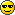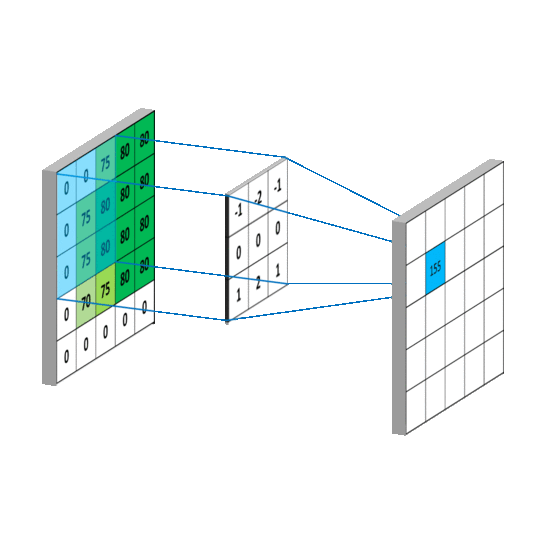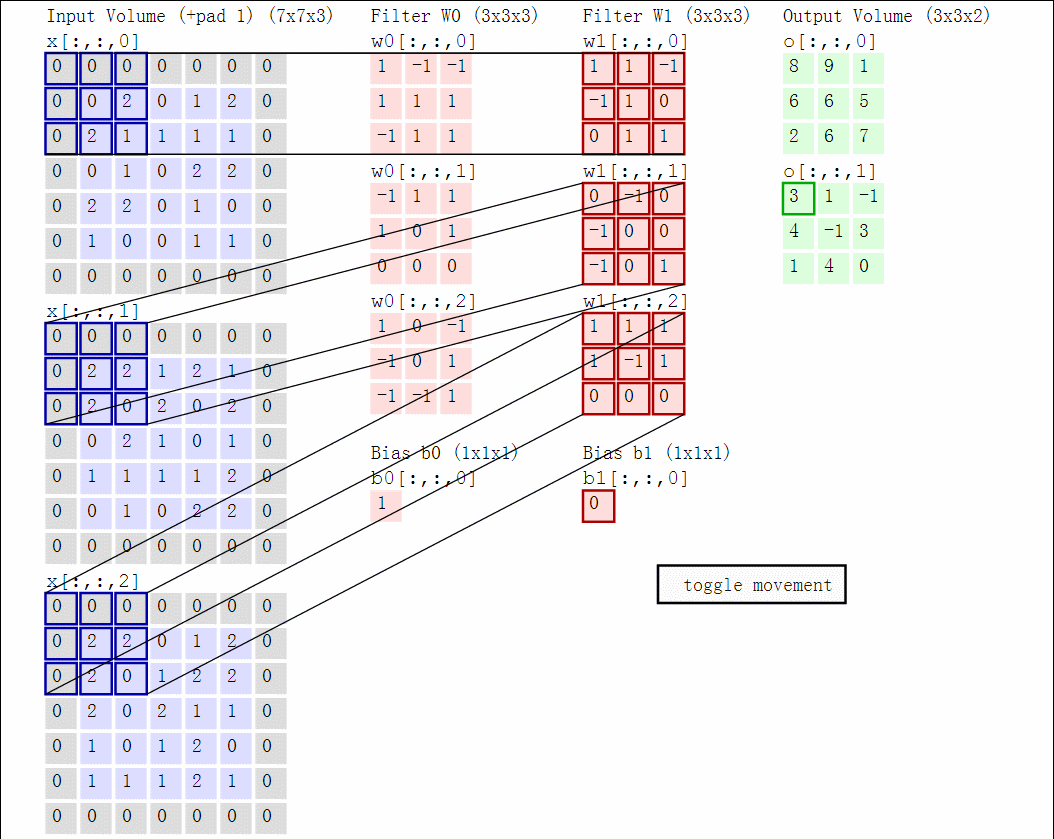Warning

# Convolutional Neural Networks

In this lab, we: (i) learn how to make a simple neural network (NN) in a pure NumPy, and (ii) build and train Convolutional Neural Networks (CNN) using the PyTorch framework. In particular, we will train several different CNN classifiers of fashion articles from 28×28 FashionMNIST grayscale images.

Today, there are many resources available about deep learning and CNNs. You can find some useful ones at the bottom of this page. However, hold on with reading them all at first. In this lab, we are going slowly. We add one component after another. There are links to relevant explanations in the text itself, so whenever you meet a new term, you also learn its meaning. You may want to revisit these links or check some other resources after finishing the lab.

## Information for development

To fulfil this assignment, you need to submit these files (all packed in a single .zip file) into the upload system:

• numpy_nn.py - containing the following implemented classes:
• ReLU - REctified Linear Unit layer
• Linear - Linear (aka Fully Connected) layer
• Sigmoid - Logistic Sigmoid layer
• SE - Squared Error loss layer
• numpy_nn_training.png, numpy_nn_classification.png
• pytorch_cnn.py - containing the following implemented classes and methods:
• MyNet - Your CNN model
• classify - classifies data using a trained network
• model.pt - trained MyNet state

Use template of the assignment. When preparing a zip file for the upload system, do not include any directories, the files have to be in the zip file root.

The challenge: The network will be automatically evaluated by the upload system and ranked in the online score board (the real-time update). The gained points from the assignment depend on the rank of your algorithm (assuming your code passes all the AE tests):

• 1st place: 16 points
• 2nd place: 15 points
• 3rd place: 14 points
• 4th place: 13 points
• 5th place: 12 points
• 6th place: 11 points
• 7th place: 10 points
• 8th place: 9 points
• every submission with performance worse than the baseline: 0 points
• 8 points otherwise

Deadline for the submission is SUNDAY 8th January 2023 23:59. Then the points will be assigned. Every later submission is then for 6 points. You have to be better than the baseline to complete this lab.

## 1. Simple Neural Network in NumPy

We start by implementing few simple layers in pure NumPy to get the understanding of what is going on in the machine learning frameworks like PyTorch, TensorFlow, etc.

Recall, that a neural network is an acyclic graph with individual nodes being either simple Perceptrons (with non-linearity) or other 'layers'. A layer is an object which has two main functionalities: (i) it can pass the data forward during the prediction/classification phase – implemented in layer.forward method, and (ii) it computes gradients with respect to (w.r.t.) its inputs which are then passed backward during back-propagation training – implemented in layer.backward method. This allows to chain many layers into possibly large graphs and then compute efficiently the gradient of the loss function w.r.t. every parameter. The forward pass propagates the input data through the forward calls in each layer sequentially from the first to the last layer. Then, starting at the last layer, the gradients are propagated backwards in the graph (with the backward function) down to all parameters. The basic example of backpropagation was given in the lecture. For a more detailed overview see e.g. the respective lecture of the Deep Learning course or the lecture by Karpathy. After all the gradients are computed, we train the network by gradient descent.

You will see that out of all layers which we consider, only the fully-connected layer (or linear layer as we call it) has some trainable parameters. During training, we update by the partial derivatives of the loss w.r.t. the parameters of the network. You might consider the parameters as another extra input of the layer (along with the outputs from the previous layer). For a layer with trainable parameters, there is also a method layer.grads in the template which should return the gradients of the loss w.r.t. its parameters.

Implement the following layers.

### Fully-Connected (Linear) layer

In the forward method, the Linear layer implements the function $f(\mathbf{x}) = \mathbf{x} \mathbf{W} + \mathbf{b}$ (This is pytorch-style definition, note that it differs from the one in lecture: Wx + b). Hint: You may need to store the inputs $x$ for later use in the backward method.

In the backward method, the gradient of the layer w.r.t. $\mathbf{W}$ and $\mathbf{b}$ is computed. The method returns the gradient of the loss w.r.t. the layer's input. Both forward and backward methods must work with several data samples stacked together (these are called batches or minibatches). Make sure that you check your shapes against the docstring specifications! Remember, CHECK YOUR SHAPES! Reshaping, adding extra dimensions or transposing the arrays may come handy (you might want to check the NumPy - HOW TO thread at the forum).

Make sure that the gradients' computation is working for batched data and their shapes are the same as the docstring tells you. If you are getting a weird error in BRUTE, it is very likely that your shapes are wrong!

input_dim, output_dim, batch_size = 3, 2, 2
linear_layer = Linear(input_dim, output_dim)
linear_layer.W = np.linspace(1, -1, input_dim * output_dim).reshape(input_dim, output_dim)
linear_layer.b = np.linspace(-0.5, 0.5, output_dim).reshape(1, output_dim)
x = np.linspace(-2, 2, batch_size * input_dim).reshape(batch_size, input_dim)
forward_output = linear_layer.forward(x)
print(f'Forward pass of your linear layer:\n{forward_output}\n')
# -> Forward pass of your linear layer:
# -> [[-2.5  -0.06]
# ->  [-1.06 -1.5 ]]

dL_wrt_output = np.linspace(1,2,output_dim * batch_size).reshape(batch_size, output_dim)
dL_wrt_x = linear_layer.backward(dL_wrt_output)
print(f'Backward pass of your linear layer:\n{dL_wrt_x}\n\n'+\
# -> Backward pass of your linear layer:
# -> [[ 1.8        -0.06666667 -1.93333333]
# ->  [ 2.86666667 -0.06666667 -3.        ]]
# ->
# -> [[[-2.         -2.66666667]
# ->   [-1.2        -1.6       ]
# ->   [-0.4        -0.53333333]]
# ->
# ->  [[ 0.66666667  0.8       ]
# ->   [ 2.          2.4       ]
# ->   [ 3.33333333  4.        ]]]
# ->
# -> [[[1.         1.33333333]]
# ->  [[1.66666667 2.        ]]]

### ReLU non-linearity

ReLU is a commonly used non-linear layer which computes $f(\mathbf{x}) = \text{max}(\mathbf{0}, \mathbf{x})$. Both forward and backward methods are much simpler as the layer has no parameters.

Make sure it works for arbitrarily shaped inputs (even 3D, 4D, or more-D)!

And again, you will need to remember what happened during the forward pass in order to compute the gradient correctly.

n_data_bw = 9
dL_wrt_output_relu = np.linspace(-1,1,n_data_bw)
x_relu = np.linspace(-1,1,n_data_bw)
relu_layer = ReLU()
_ = relu_layer.forward(x_relu)
dL_wrt_x_relu = relu_layer.backward(dL_wrt_output_relu)
print(f'Backward pass of your ReLU layer:\n{dL_wrt_x_relu}')
# -> Backward pass of your ReLU layer:
# -> [0.   0.   0.   0.   0.   0.25 0.5  0.75 1.  ]

### Sigmoid non-linearity

You already know the logistic sigmoid from the logistic regression lab. The Sigmoid layer implements the function $f(\mathbf{x}) = \frac{1}{1 + e^{-\mathbf{x}}}$. As in the ReLU layer, there are no parameters in the Sigmoid layer. The backward method only computes the gradient of the loss w.r.t. the layer inputs.

n_data_bw = 5
dL_wrt_output_sigmoid = np.linspace(-10,10,n_data_bw)
x_sigmoid = np.linspace(-5,5,n_data_bw)
_ = sigmoid_layer.forward(x_sigmoid)
dL_wrt_x_sigmoid = sigmoid_layer.backward(dL_wrt_output_sigmoid)
print(f'Backward pass of your Sigmoid layer:\n{dL_wrt_x_sigmoid}')
# -> Backward pass of your Sigmoid layer:
# -> [-0.06648057 -0.35051858  0.          0.35051858  0.06648057]

### Squared Error Loss

The squared error loss computes $(\mathbf{x}-\mathbf{y})^2$. The backward pass computes the gradient of the loss only w.r.t. the input $x$.

n_data_bw = 9
dL_wrt_output_se = np.linspace(-10,10,n_data_bw)
x_se = np.linspace(-5,5,n_data_bw)
_ = se_layer.forward(x_se, 0.5*x_se)
dL_wrt_x_se = se_layer.backward()
print(f'Backward pass of your Squared Error Loss layer:\n{dL_wrt_x_se}')
# -> Backward pass of your Squared Error Loss layer:
# -> [-5.   -3.75 -2.5  -1.25  0.    1.25  2.5   3.75  5.  ]

## Defining and training the network

Your task is to train a network for a binary classification of two selected classes (numbers) from the MNIST dataset. The data loading, the model definition, and training is done in the main() method in the section of the numpy_nn.py template or in the second part of the jupyter notebook numpy_nn.ipynb.

Experiment with the hyper-parameter settings (Slow training? Try increasing learning_rate. No convergence? Try decreasing learning_rate) and the model architecture. A typical model has several layers organised as Linear → ReLU → Linear → ReLU → … → Linear → Sigmoid, but many other options are possible.

1. Does adding more fully-connected layers help?
2. Does having more output units in the fully-connected layer help?
3. What activation functions work the best?
4. Experiment with the batch size and watch how it influences the training.
5. Feel free to implement also other layers and non-linearities.

Save the training images as numpy_nn_training.png and numpy_nn_classification.png

## 2. PyTorch Network

Our simple NumPy NN works well for a simple two digit classification problem, but if we want to solve a more difficult task, it quickly becomes inefficient and then it is better to use one of the highly optimized public libraries. We will work with the currently most popular CNN framework - PyTorch. Writing the neural network using PyTorch is straightforward - there are many layers readily available and you can operate on the data passing through the network just like you would in plain NumPy.

A template for this part of the assignment is in pytorch_cnn.py.

## Introduction to PyTorch

Working with PyTorch should not feel much different from what we just did in NumPy, except all the layers are already implemented for you. Reading through the pytorch_cnn.py in the template should give you a good initial idea about PyTorch basics.

We also recommend the documentation (e.g. PyTorch 1.12 Conv2d documentation) and the official tutorials:

• Official PyTorch introduction course. There are step-by-step tutorials, documentation and a possibility of using Google Colab notebooks for learning PyTorch.

## PyTorch Instalation

Start by installing PyTorch (most likely cpu-only version) - follow the instructions at https://pytorch.org/get-started/locally/.
For example (conda):

conda install pytorch==1.12.1 torchvision==0.13.1 cpuonly -c pytorch

or (pip):

pip install torch==1.12.1+cpu torchvision==0.13.1+cpu --extra-index-url https://download.pytorch.org/whl/cpu

Only use the PyTorch features compatible with the PyTorch version used by BRUTE.

## Problem specification

Your task is to classify images of fashion articles. Fortunately, there is quite a lot of annotated data readily available on the internet: FashionMNIST. To simplify loading the data, use torchvision FashionMNIST dataset which downloads and prepares the data for you (see the template).

Unfortunately, during the testing phase, your customer (a company called BRUTE & SON) is using a private collection of real-world images. The testing set has the same classes and similar statistics as the training data. Nevertheless, even though the style of article images in FashionMNIST dataset is representative enough, the test images are not from FashionMNIST test subset.

If you intend pass this lab only, you should be fine with custom architecture and 10% of FashionMNIST dataset in your training. However, if you want to earn some bonus points by training the best performing network, you should use the data augmentation technique and make your network robust to brightness and color shifts expected in the real-world data. We have tried with FashionMNIST data only and things did not go well. That's why we are hiring you after all ;)

Examples of FashionMNIST data:

### 2.1 Linear classifier, multinomial logistic regression, stochastic gradient descent

Lets start with a simple one layer fully-connected network. You should now understand how the backpropagation works under the hood after the first part of this assignment. Here we will need to extend the binary classification to the multi-class one. Fortunately, this is quite easy using the multinomial logistic regression model. Simply, the predictive probabilities of classes are computed not by sigmoid but by softmax:

$$p(y{=}k|s) = {\rm softmax}(s)_k = \frac{e^{s_k}}{\sum_j e^{s_j}},$$

where $s$ is a vector of scores (one per class) computed by the preceding layers of the network. For the purpose of numerical stability it is convenient to adopt the convention that the network should output log probabilities, and use the function log_softmax as in the template.

The template contains a code for training a simple one-layer network FCNet with a log-softmax output. It is trained using the stochastic gradient descent to minimize the negative log likelihood loss (NLL).

1. Train the FCNet network on FashionMNIST data (see the train function in the template)

2. Add another fully-connected layer with 1000 hidden units and with sigmoid non-linearity. Do you get better results?

3. Try to add more layers. Does the network improve with more and more layers?

4. How many weights do you learn in each case?

5. Try to substitute the sigmoid non-linearity with Rectified Linear Unit (ReLU). It helps to avoid the vanishing gradient problem.

6. Experiment with the number of layers, number of hidden units and try to get the best possible result.

### 2.2 Convolutional Neural Networks

One of the main disadvantages of using the fully-connected layers on images is that they do not take into account the spatial structure of the image. Imagine that you randomly perturb spatial arrangement of image pixels (in both training and test data) and re-train the fully-connected network. These perturbed images become completely unlearnable for humans, since humans make a prior assumption about the spatial arrangement. Nevertheless, the expected testing error of the re-trained network on this randomly perturbed dataset will be the same, since it does not make any assumptions and learns the spatial arrangement from the scratch from perturbed training data. When we learn on images, architecture of the network should reflect the particular spatial arrangement.

We impose the spatial arrangement by introducing the convolutional layers. The convolution works as shifting a local template (often called a convolution kernel or a local receptive field) over the image and computing its response for every single position in the image. For example, when the input image is 28×28, and we compute convolution with 5×5 kernel, then the resulting response image will be 24×24 (unless we pad the image with zeros). When learned, these templates often corresponds to edge or corner detectors.

Illustration for 3×3 kernel, single input and single output channel (source):Illustration for 3×3 kernel, padding 1, stride 2, three input and two output channels (source):Another disadvantage of the fully-connected layers is that the number of parameters grows quickly with new layers. This means significantly more parameters need to be learned and thus more data need to be used to avoid overfitting.

1. Train a CNN with one convolutional layer (3×3 kernel) followed by a ReLU non-linearity and a fully connected layer with log-softmax output (see SimpleCNN provided in the template).

Notice that the kernel is not evaluated at every position but at every second only (stride=2). This makes the second layer smaller while keeping most of the information present (as nearby convolutions result in similar values). We also added padding=1, which adds zeros to the image before computing the convolution. This way, the size of the output stays the same when stride=1 and becomes half when stride=2.

2. Are you getting better results than with fully connected networks? Does taking into account the spatial arrangement help?

3. How many parameters are you learning now? Compare this to the case of the two layered fully connected network.

4. Add one more convolutional layer with ReLU (again with stride=2).

5. Visualise the learned filters in the first convolutional layer (they are 3×3 matrices).

6. To train a CNN one still needs a lot of data as the number of parameters being estimated is large. To avoid over-fitting, two another techniques are commonly used: max-pooling and dropout. Substitute the stride=2 by stride=1 and max-pooling and add a dropout layer before the fully connected layer.

7. In order to overcome the baseline performance and get 8 points implement your network in MyNet - playing with the following techniques should be sufficient:
8. For bonus points: Experiment! Your goal is to train the best possible network, submit it to the upload system for the competition. You can play with (in random order):

Beware! You are supposed to fine-tune the network using your validation set. The number of uploads is limited to 100 attempts by BRUTE, but try to keep it even smaller. If we see unreasonably many uploads for one student, the network could be disqualified as over-fitted to the test data!

Note that some students report a better user experience when training their pytorch network in Google Colab.